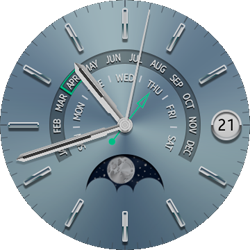# Special Edition Watch Sample Overview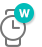Related Info

The Special Edition Watch sample application demonstrates how to implement the Tizen official Special Edition Watch face application using the Tizen Time API.

The following figure illustrates the main screen of the Special Edition Watch.

Figure: Special Edition Watch screen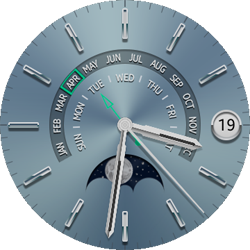The application opens with the main screen that shows the current time and date.

Moreover, the watch face application shows the current moon phase.

## Source Files

You can create and view the sample application project including the source files in the IDE.

File name Description
config.xml This file contains the application information for the platform to install and launch the application, including the view mode and the icon to be used in the device menu.
css/style.css This file contains the CSS styling for the application UI.
index.html This is a starting file from which the application starts loading. It contains the layout of the application screens.
js/app.js This file starts the application.
js/moonphase.js This file calculates the angle that is used to rotate the moon image.

## Implementation

### Application Layout

The application has only 1 screen, whose content is defined in the index.html file.

There are 4 sections for the date number, hand, calendar, and moon phase.

```<!--Body section-->
<div id="body-bg">

<!--Date number section-->
<div id="body-date-num">
<div id="date-num-string"></div>
</div>

<!--Hand section-->
<div id="body-sec-hand" class="sec-hand"></div>
<div id="body-min-hand" class="min-hand"></div>
<div id="body-hr-hand" class="hr-hand"></div>

<!--Calendar section-->
<div id="body-calendar-unit"></div>
<div id="body-calendar-stroke"></div>
<div id="body-calendar-hand" class="calendar-hand"></div>

<!--Moon phase section-->
<div id="body-moonphase">
<div id="moonphase-cover"></div>
<div id="moonphase-moon"></div>
<div id="moonphase-nightsky"></div>
</div>

</div>```

### Displaying the Moon Phase Images

This application uses 3 images for the moon phase functionality.

Figure: Images for the Moonphase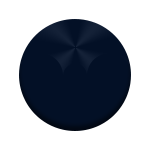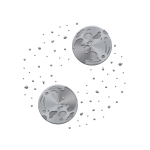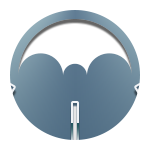Put the first image on the lowest layer and the third image at the top. The second image is put in between the first and the third images. Only the second image rotates at the calculated angle for moon phase function.

Use the z-index attribute to put the 3 images on top of each other. The element with a higher value of the z-index is put on top of those with lower z-index values.

```<!--style/style.css-->
#moonphase-nightsky
{
position: absolute;
width: 100%;
height: 100%;
z-index: 1;
}

#moonphase-moon
{
position: absolute;
width: 100%;
height: 100%;
z-index: 2;
}

#moonphase-cover
{
position: absolute;
width: 100%;
height: 100%;
z-index: 3;
}
```

### Calculating the Rotating Angle for the Moon Phase

In this application, the new moon is defined as the standard point to calculate the rotating angle.

To calculate the rotating angle:

1. The rotating angle for the new moon in the images is 60 degrees.

```/* js/app.js */

var NEW_MOON_ANGLE = 60;
```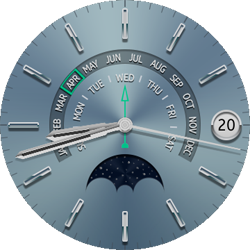2. Using the current date, find the current timestamp in the moon phase calendar and calculate the angle to rotate.

Add the angle and the standard point angle for the new moon.

The final rotating angle is calculated by adding the NEW_MOON_ANGLE (60 degrees) and the angle for the current moon phase status.

```/* js/app.js */
/* Parameter of moonphase.inputDate() is date information, it returns the rotate angle based on the new moon */
rotateAngle = moonphase.inputDate(dateInformation);
rotateElement(NEW_MOON_ANGLE + rotateAngle, "moonphase-moon");
```
3. This application uses the timestamp of the moon phase calendar to calculate the rotating angle.

Add the timestamp data of the moon phase calendar to an array.

This sample adds the timestamp data for only 5 years (2016-2020). For example, it can check the 2016 data array set as below.

```/* js/moonphase.js */
/* 2016 data set */
/* First phase stamp is "Last Quarter" */

MOON_PHASE_DATA =
[
"0102","0110","0116","0124",
"0201","0208","0215","0222",
"0301","0309","0315","0323","0331",
"0407","0414","0422","0430",
"0506","0513","0521","0529",
"0605","0612","0620","0627",
"0704","0712","0719","0726",
"0802","0810","0818","0825",
"0901","0909","0916","0923",
"1001","1009","1016","1022","1030",
"1107","1114","1121","1129",
"1207","1214","1221","1229"
],
```
4. For each year, set the first stamp to an array.

For example, the first timestamp of 2016 is last quarter.

```/* js/moonphase.js */

/*
For each year, set the first stamp (2016-2020)
0: New moon
1: First quarter
2: Full moon
3: Last quarter
@type {number[]}
*/

MOON_PHASE_FIRST_ANGLE =
[
3, 1, 2, 0, 1
],
```
5. Find the current timestamp from the MOON_PHASE_DATA array using the input date information. Then, set the current timestamp and the next one in the setCurrentStamp() method.

For example, suppose the current date is April 21, 2016. Find where the current timestamp exits in the MOON_PHASE_DATA array. The start stamp is "0414", and the last stamp in that array is "0422".

In this example (April 21, 2016), the start stamp is first quarter and the last stamp is full moon. In other words, current moon phase is in the waxing status from the first quarter to the full moon.

```/* js/moonphase.js */
function setCurrentStamp()
{
var i;

startStamp = lastStamp = "NULL";

/* Find the stamp using the current date */
for (i=0; i<MOON_PHASE_DATA[year].length; i++ )
{
/* If the stamp date equals the current date, set the current stamp to that stamp */
if (MOON_PHASE_DATA[year][i] === fullDate)
{
startStamp = lastStamp = MOON_PHASE_DATA[year][i];
currentStampNumber = (MOON_PHASE_FIRST_ANGLE[year] + i) % 4;
break;
}

/* If it is not, find the median stamp */
else if (MOON_PHASE_DATA[year][i] > fullDate)
{
/* [year][i-1] ~ [year][i] */
startStamp = MOON_PHASE_DATA[year][i-1];
lastStamp = MOON_PHASE_DATA[year][i];

/*
If today is smaller than the first stamp,
set startStamp as the last stamp of the previous year
*/
if (i === 0)
{
startStamp = MOON_PHASE_DATA[year-1][MOON_PHASE_DATA[year-1].length - 1];
lastStamp = MOON_PHASE_DATA[year][i];
}

currentStampNumber = (MOON_PHASE_FIRST_ANGLE[year] + i + 3) % 4;
break;
}
}

/* There is no stamp in the current year, set the first stamp of the next year */
if (startStamp === "NULL" && lastStamp === "NULL")
{
startStamp = MOON_PHASE_DATA[year][MOON_PHASE_DATA[year].length - 1];
lastStamp = MOON_PHASE_DATA[year+1];
currentStampNumber = (MOON_PHASE_FIRST_ANGLE[year] + i + 3) % 4;
}
}```
6. To determine how much is left for the Moon to become full, the total days in between the start stamp and the last stamp as well as the passing days are calculated in the CalcAngle() method.

• The denomiStamp is 9 ("4022"-"4014") as total days in between the start stamp and the last stamp.
• The numerStamp is 8 ("0421"-"0414") as passing days from the start stamp to the current date.

Using these values, calculate the angle for rotating the second image:

angle = (8/9)*45 + 45 * 1(first quarter)

```/* js/moonphase.js */
function calcAngle()
{
var denomiStamp = lastStamp - startStamp + 1,
numerStamp = fullDate - startStamp + 1,
startSubMonth = parseInt(startStamp.substring(0, 2), 10),
startSubDay = parseInt(startStamp.substring(2, 4), 10),
lastSubDay = parseInt(lastStamp.substring(2, 4), 10),
todaySubDay = parseInt(fullDate.substring(2, 4), 10),
startYearMonthDay;

/* If current year is leap year */
if (isLeapYear(year))
{
startYearMonthDay = LEAP_YEAR_DAY[startSubMonth-1];
}
else
{
startYearMonthDay = YEAR_DAY[startSubMonth-1];
}

if (denomiStamp > MINIMUM_DAY_DIFFERENCE || denomiStamp < 0)
{
denomiStamp = (startYearMonthDay - startSubDay) + 1 + lastSubDay;
}

if (numerStamp > MINIMUM_DAY_DIFFERENCE || numerStamp < 0)
{
numerStamp = (startYearMonthDay - startSubDay) + 1 + todaySubDay;
}

if (startStamp === lastStamp)
{
angle = 45 * currentStampNumber;
}
else
{
angle = (numerStamp / denomiStamp * 45) + 45 * currentStampNumber;
}
}```

The following figure shows the moon phase for April 21, 2016.